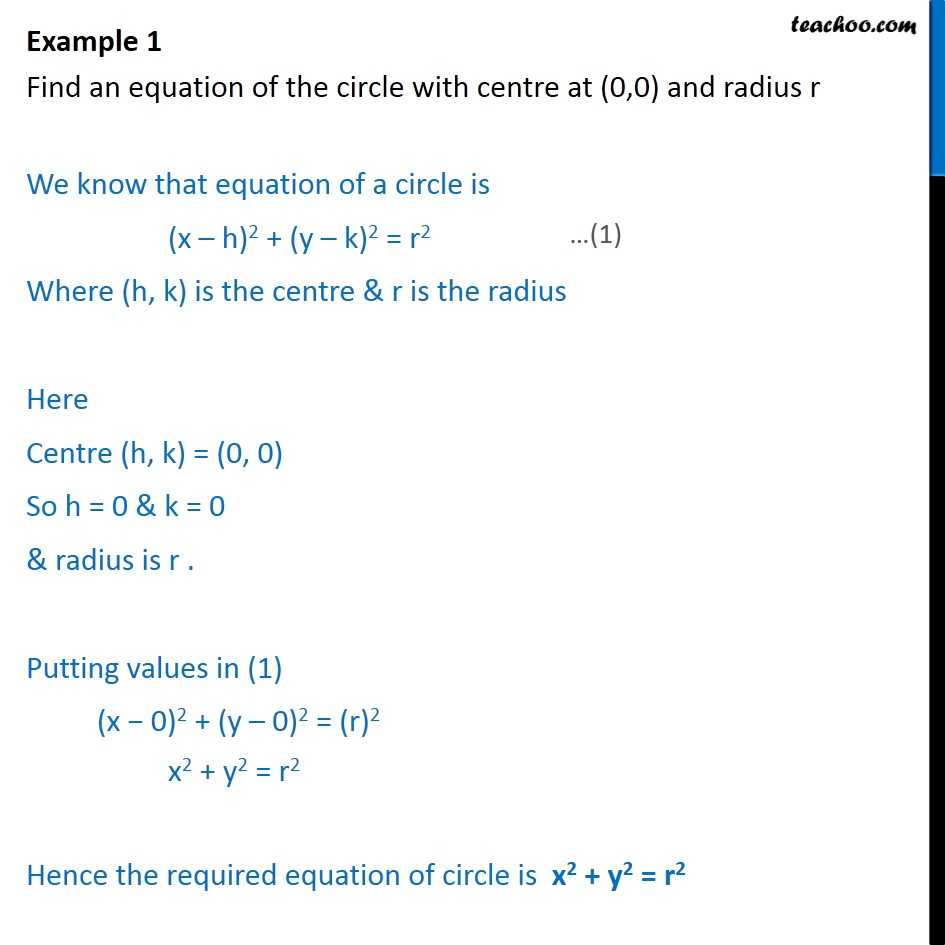Examples

Chapter 10 Class 11 Conic Sections
Serial order wiseLearn in your speed, with individual attention - Teachoo Maths 1-on-1 Class

### Transcript

Example 1 Find an equation of the circle with centre at (0,0) and radius r We know that equation of a circle is (x­ – h)2 + (y ­– k)2 = r2 Where (h, k) is the centre & r is the radius Here Centre (h, k) = (0, 0) So h = 0 & k = 0 & radius is r . Putting values in (1) (x − 0)2 + (y – 0)2 = (r)2 x2 + y2 = r2 Hence the required equation of circle is x2 + y2 = r2